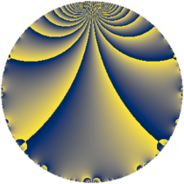# Properties

 Label 21.2.eLevel $21$ Weight $2$ Character orbit 21.e Rep. character $\chi_{21}(4,\cdot)$ Character field $\Q(\zeta_{3})$ Dimension $2$ Newform subspaces $1$ Sturm bound $5$ Trace bound $0$

# Related objects

## Defining parameters

 Level: $$N$$ $$=$$ $$21 = 3 \cdot 7$$ Weight: $$k$$ $$=$$ $$2$$ Character orbit: $$[\chi]$$ $$=$$ 21.e (of order $$3$$ and degree $$2$$) Character conductor: $$\operatorname{cond}(\chi)$$ $$=$$ $$7$$ Character field: $$\Q(\zeta_{3})$$ Newform subspaces: $$1$$ Sturm bound: $$5$$ Trace bound: $$0$$

## Dimensions

The following table gives the dimensions of various subspaces of $$M_{2}(21, [\chi])$$.

Total New Old
Modular forms 10 2 8
Cusp forms 2 2 0
Eisenstein series 8 0 8

## Trace form

 $$2 q - 2 q^{2} - q^{3} - 2 q^{4} + 2 q^{5} + 4 q^{6} - 5 q^{7} - q^{9} + O(q^{10})$$ $$2 q - 2 q^{2} - q^{3} - 2 q^{4} + 2 q^{5} + 4 q^{6} - 5 q^{7} - q^{9} + 4 q^{10} + 2 q^{11} - 2 q^{12} + 2 q^{13} + 2 q^{14} - 4 q^{15} + 4 q^{16} - 2 q^{18} - q^{19} - 8 q^{20} + 4 q^{21} - 8 q^{22} + q^{25} - 2 q^{26} + 2 q^{27} + 8 q^{28} + 8 q^{29} + 4 q^{30} - 9 q^{31} + 8 q^{32} + 2 q^{33} - 2 q^{35} + 4 q^{36} - 3 q^{37} - 2 q^{38} - q^{39} - 20 q^{41} - 10 q^{42} + 10 q^{43} + 4 q^{44} + 2 q^{45} + 6 q^{47} - 8 q^{48} + 11 q^{49} - 4 q^{50} - 2 q^{52} - 12 q^{53} - 2 q^{54} + 8 q^{55} + 2 q^{57} - 8 q^{58} + 12 q^{59} + 4 q^{60} - 10 q^{61} + 36 q^{62} + q^{63} - 16 q^{64} + 2 q^{65} + 4 q^{66} + 5 q^{67} - 16 q^{70} - 12 q^{71} + 3 q^{73} - 6 q^{74} + q^{75} + 4 q^{76} - 8 q^{77} + 4 q^{78} + q^{79} - 8 q^{80} - q^{81} + 20 q^{82} + 12 q^{83} + 2 q^{84} - 10 q^{86} - 4 q^{87} - 16 q^{89} - 8 q^{90} - 5 q^{91} - 9 q^{93} + 12 q^{94} + 2 q^{95} + 8 q^{96} - 12 q^{97} + 4 q^{98} - 4 q^{99} + O(q^{100})$$

## Decomposition of $$S_{2}^{\mathrm{new}}(21, [\chi])$$ into newform subspaces

Label Dim $A$ Field CM Traces $q$-expansion
$a_{2}$ $a_{3}$ $a_{5}$ $a_{7}$
21.2.e.a $2$ $0.168$ $$\Q(\sqrt{-3})$$ None $$-2$$ $$-1$$ $$2$$ $$-5$$ $$q+(-2+2\zeta_{6})q^{2}-\zeta_{6}q^{3}-2\zeta_{6}q^{4}+\cdots$$